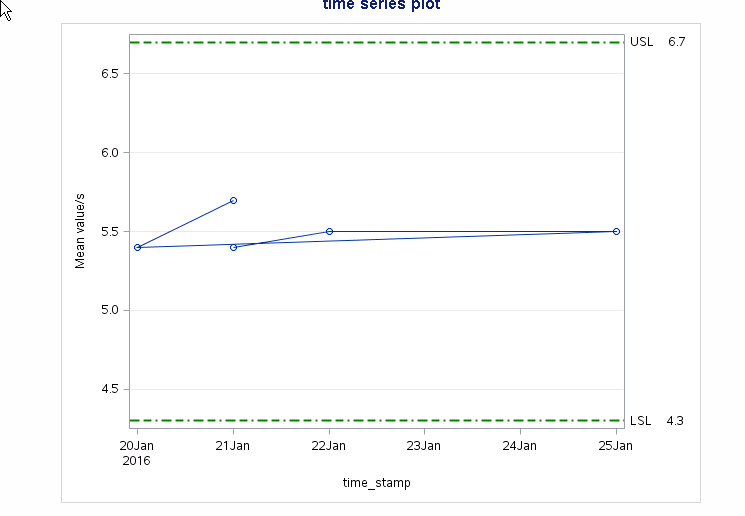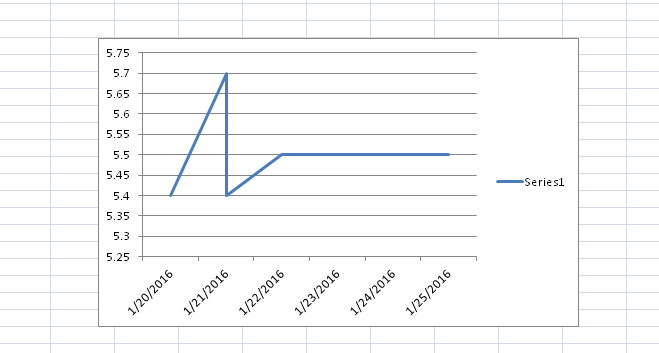## Time series using Proc sgplot

Hello,

I am trying to create timeseries graph using proc sgplot but in my case there are multiple records available for single date.this causes following graph to be formed.Here i have two rows for 21 jan .instead i would like to make graph like this:Is there any way to get 2nd graph?

I am using this code:

proc sgplot data=work.limits;
series x=time_stamp y=test_value/markers ;
refline &bin_lsl/axis=Y label= 'LSL&bin_lsl.' lineattrs=(color ='green' thickness=2 pattern =dashdashdot);
refline &bin_usl/axis=Y label= 'USL&bin_usl.' lineattrs=(color ='green' thickness=2 pattern =dashdashdot);
xaxis discreteorder=data fitpolicy=rotate display=all;
yaxis grid max=&bin_usl min = &bin_lsl ;

1 ACCEPTED SOLUTION

Accepted Solutions

## Re: Time series using Proc sgplot

You need to sort the data by the X variable.  There is not a unique way to plot data that has duplicate X values, but in your example you are plotting the high value before the low values, so run the following before you call PROC SGPLOT:

``````proc sort data=work.limits;
by time_stamp test_value;
run;``````
2 REPLIES 2

## Re: Time series using Proc sgplot

Remove duplicate records before doing the graph?

## Re: Time series using Proc sgplot

You need to sort the data by the X variable.  There is not a unique way to plot data that has duplicate X values, but in your example you are plotting the high value before the low values, so run the following before you call PROC SGPLOT:

``````proc sort data=work.limits;
by time_stamp test_value;
run;``````
Discussion stats
• 2 replies
• 2409 views
• 2 likes
• 3 in conversation